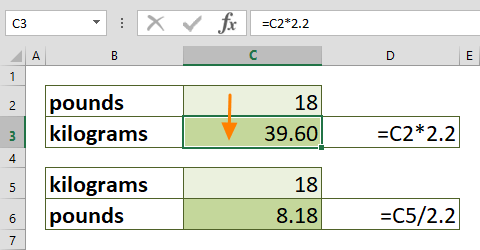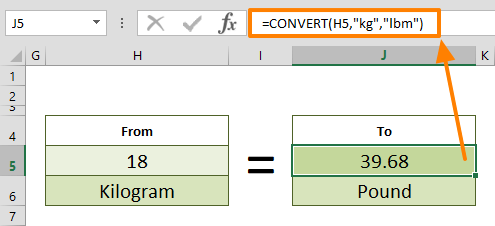Converting units can be time consuming when you rely on a separate tool or website to do it. In this guide, we are going to show you how to convert pounds to kg and kg to pounds.

## Manual approach

The first approach we are going to show you is doing the conversion manually. You can convert pounds to kg with a simple math operation. 1 kg is approximately 2.2 lbs. Thus, you need to divide the pound-value by 2.2 to get the value in kilograms.

Let's say the cell C2 contains the pound value we want to convert. Use a formula like below to find the kilogram value.

=C2/2.2In a similar way, you can convert kg to pounds. As shown in the above example, multiplying a kilogram-value by 2.2 returns the value in pounds. If we assume that the cell C5 contains the kilogram-value, the conversion formula would be,

=C5*2.2

Now, let’s take a look at more dynamic and relatively easy to remember approaches.

## Using the CONVERT Function

Another way to convert pounds to kg is using the CONVERT function. The CONVERT function can convert a number from one measurement system to another. The best part is that the function is not limited to only length units.  You can convert length, distance, temperature and more units of measurements between each other.

You can find more information about the CONVERT function with samples in our guide: Excel CONVERT Function

Briefly, you need to enter both the value and measurement types into the function. Excel accepts string abbreviations of the measurement types. In this example you can use,

• Kilograms: "kg"
• Pounds: "lbm"

As a result, to convert the value in cell B5, you need to use the formula below:

=CONVERT(B5,"lbm","kg")To make the conversion in the opposite direction, simply switch the measurement arguments. The following formula converts the value from pounds to kg.

=CONVERT(B5,"kg","lbm")## Another dynamic method

You can set cell references for unit arguments. This application allows you to control the from and to unit types. We combined the CONVERT and VLOOKUP functions to create a master formula which gets units to convert from a list or any other input type. With the help of the VLOOKUP function, we can use user-friendly names for unit type codes. For example, "pounds" for "lbm" and "kilogram" for "kg".

The VLOOKUP returns the corresponding unit type for the CONVERT function. Here is what the formula can look like:

=CONVERT(B11,VLOOKUP(B12,Mass.Table,2,0),VLOOKUP(D12,Mass.Table,2,0))

The cells B12 and D12 contains a dropdown list which contains mass type of measurement units like "Pound" and "Kilogram" strings. The VLOOKUP searches them in the named range Mass.Table and returns the corresponding value.